Courses

# Work, Power And Energy MSQ

## 10 Questions MCQ Test Basic Physics for IIT JAM | Work, Power And Energy MSQ

Description
This mock test of Work, Power And Energy MSQ for Physics helps you for every Physics entrance exam. This contains 10 Multiple Choice Questions for Physics Work, Power And Energy MSQ (mcq) to study with solutions a complete question bank. The solved questions answers in this Work, Power And Energy MSQ quiz give you a good mix of easy questions and tough questions. Physics students definitely take this Work, Power And Energy MSQ exercise for a better result in the exam. You can find other Work, Power And Energy MSQ extra questions, long questions & short questions for Physics on EduRev as well by searching above.
*Multiple options can be correct
QUESTION: 1

### One end of an unstretched vertical spring is attached to the ceiling and an object attached to the other end is slowly lowered to its equilibrium position. If S be gain in spring energy and G be loss in gravitational potential energy in the process, then which of the following is false.

Solution: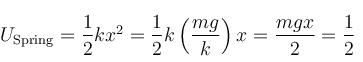(loss in gravitationl potential energy)
⇒ G = 2S
The correct answers are: S = GS = 2GS = 3G

*Multiple options can be correct
QUESTION: 2

### A block of mass 2kg is hanging over a smooth and light pulley through a light string. The other end of the string is pulled by a constant force F = 40N. The kinetic energy of the particle increase 40J in a given interval of time. Then : (g = 10m/s2)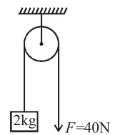Solution:

Free body diagram of block is as shown in figure.
From work-energy theorem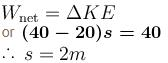Work done by gravity is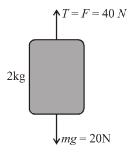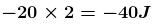and work done by tension is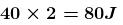The correct answers are: tension in the string is 40N, displacement of the block in the given interval of time is 2m, work done by tension is 80J

QUESTION: 3

### For a particle moving on a straight line the variation of acceleration with time is given by the graph as shown initially the particle was at rest. Then the corresponding kinetic energy of the particle versus time graph will be :

Solution:

The above graphs show v-t graph from a-t graph and then v2-t graph, which are self explanatory.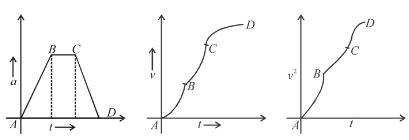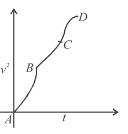*Multiple options can be correct
QUESTION: 4

The potential energy for a force field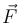is given by U(xy) = cos(x + y). The force is acting on a particle at position given by coordinates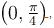then which of the following statement is true.

Solution: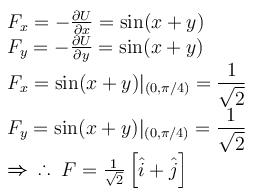The correct answers are: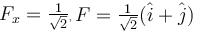*Multiple options can be correct
QUESTION: 5

There are two massless springs A and B of spring constant KA and KB respectively and KA > KB. If WA and WB be denoted as work done on A and work done on B respectively, then :

Solution:

If the springs are compressed to same amount :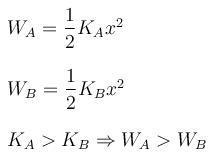If the springs are compressed by same force.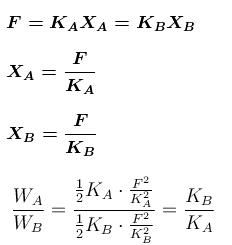The correct answers are: If they are compressed to same distance, WA > WB, If they are compressed by same force (upto equilibrium state) WA < WB

*Multiple options can be correct
QUESTION: 6

Which of the following is/are conservative force(s)?

Solution: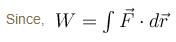Clearly for forces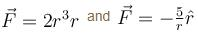the integration do not require any information of the path taken.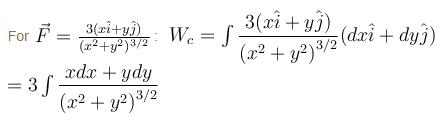Taking : x2 + y2 = t
2xdx + 2ydy = dt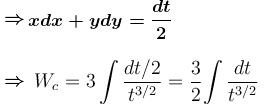which is solvable.

Hence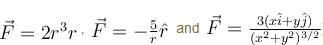are conservative forces.

But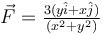The correct answers are: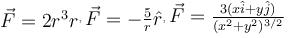*Multiple options can be correct
QUESTION: 7

The potential energy (in Joules) of a particle of mass 1kg moving in a plane is given by V = 3x + 4y, the position coordinates of the point being x and y, measured in metres. If the particle is at rest at (6,4); then

Solution: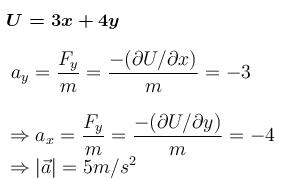Let at time 't' particle crosses y-axis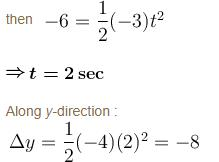⇒ Particle crosses y-axis at y = –4
At (6, 4) U = 34 & KE = 0
At (0, 4); U = –16
⇒ KE = 50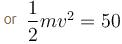⇒ v = 10 m/s  while crossing y-axis
The correct answers are: its acceleration is of magnitude 5m/s2, its speed when it crosses the y-axis is 10m/s, it crosses the y-axis (x = 0) at y = –4

*Multiple options can be correct
QUESTION: 8

In the figure shown the potential energy U of a particle is plotted against its position x  from origin. Then which of the following statement is incorrect.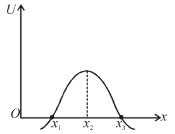Solution:

x - x1   and  x = x3  are not equilibrium positions because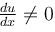at these points. x = x2 is unstable, as U is maximum at this point.

The correct answers are: x1 is in stable equilibrium, x3 is in stable equilibrium, x2 is in unstable equilibrium

*Multiple options can be correct
QUESTION: 9

Work done by a force on an rigid object having no rotational motion will be zero, if :

Solution:

(a) If velocity and acceleration are not in same direction, work done by force perpendicular to acceleration will not be zero.
(b) If the object is at rest no force can do work
(c) If force is perpendicular to velocity work done will be zero
(d) If the point on the body has velocity component in direction of application of force work done will be non-zero.

The correct answers are: the object is at rest relative to ground but the point of application of force moves on the object, the force is always perpendicular to velocity of object

*Multiple options can be correct
QUESTION: 10

An engine can pull 4 coaches at a maximum speed of 20m/s. Mass of the engine is twice the mass of every coach. Assuming resistive force to be proportional to the weight. (Power of engine remains constant) :

Solution:

When 4 coaches (m each) are attached with engine (2m)
according to questions P = K 6mgv    ...(1)
(constant power), (K being proportionality constant)
Since resistive force is proportional to weight
Now if 12 coaches are attached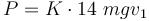...(2)

Since engine power is constant
So by equation (1) and (2)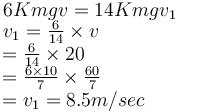Similarly for 6 coaches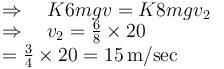The correct answers are: maximum speed of engine when it pulls 12 coaches is 8.5m/s, maximum speed of engine when it pulls 6 coaches is 15m/s